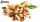Sales stores

The first sales store passed the 1/3 and the second 2/5 of the total amount of goods.In third store passed the 2/3 rest of the goods. The remaining 40 kg of goods put into fourth store. How many kilograms of goods pass to a third store?

Result

x =  80 kg

Solution:1/3y = 40
2/3y = x

y = 120
3x-2y = 0

x = 80
y = 120

Calculated by our linear equations calculator.

Leave us a comment of this math problem and its solution (i.e. if it is still somewhat unclear...):Be the first to comment!To solve this verbal math problem are needed these knowledge from mathematics:

Need help calculate sum, simplify or multiply fractions? Try our fraction calculator. Do you have a linear equation or system of equations and looking for its solution? Or do you have quadratic equation?

Next similar math problems:

1. Cleaning windowsCleaning company has to wash all the windows of the school. The first day washes one-sixth of the windows of the school, the next day three more windows than the first day and the remaining 18 windows washes on the third day. Calculate how many windows ha
2. Three monksThree medieval monks has task to copy 600 pages of the Bible. One rewrites in three days 1 page, second in 2 days 3 pages and a third in 4 days 2 sides. Calculate for how many days and what day the monks will have copied whole Bible when they begin Wednesd
3. Bag of peanutsJoe eat 1/3 of a bag of peanuts, mark eat 1/4 of the remaining in the bag of peanuts, Alvin eat 1/2 of the remaining bag of peanuts, peter eat 10 peanuts, there are 71 peanuts left. Hon many peanuts were in the bags?
4. Unknown numberI think the number - its sixth is 3 smaller than its third.
5. EquationSolve the equation: 1/2-2/8 = 1/10; Write the result as a decimal number.
6. Simple equation 6Solve equation with one variable: X/2+X/3+X/4=X+4
7. UN 1If we add to an unknown number his quarter, we get 210. Identify unknown number.
8. Unknown numberIdentify unknown number which 1/5 is 40 greater than one tenth of that number.
9. Fraction + eqSolve following simple equation with fractions: -5/6(8+5b) = 75 + 5/3b
10. Equation with mixed fractions2 3/5 of 1430+? = 1900. How to do this problem
11. Eq-fracSolve the following equation with fractions: h + 1/3 =5/3
12. Equation with xSolve the following equation: 2x- (8x + 1) - (x + 2) / 5 = 9
13. CandyPeter had a sachet of candy. He wanted to share with his friends. If he gave them 30 candies, he would have 62 candies. If he gave them 40 candies, he would miss 8 candies. How many friends did Peter have?
14. One thirdIf 3/5 is 360, how much is 1/3?
15. EqnSolve equation with fractions: 2x/3-50=40+x/4
16. Simple equationSolve for x: 3(x + 2) = x - 18
17. PearsThere were pears in the basket, I took two-fifths of them, and left six in the basket. How many pears did I take?